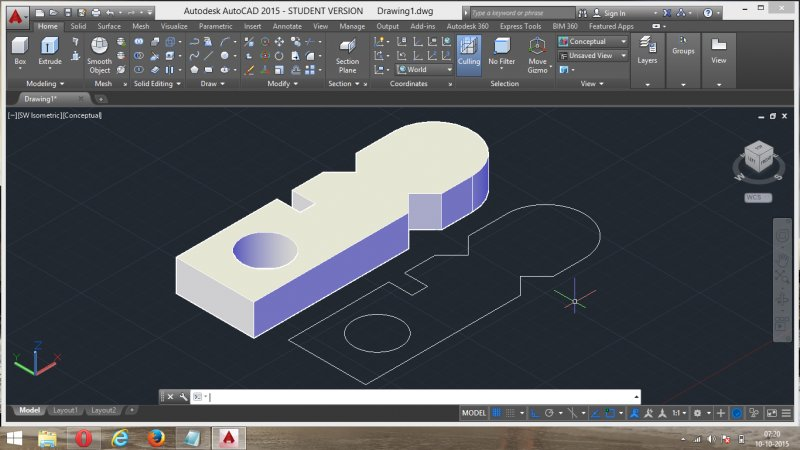# extract all coordinate and dimension from autocad drawing## Recommended Postsi want to extract all coordinate and dimension from AutoCAD drawing(attached file)

i am using below code they extract only circle dimension, what can i do to extract other dimension..(defun c:test (/ i e p1 p2 ss lst q var f fn dat dat1)

;hanhphuc 2014

(set 'var (getvar 'cmdecho ))

(setvar 'cmdecho 0)

(if (and (setq e (entsel "\nPlease select solid.. ")) (setq e (car e)) (= (cdr (assoc 0 (entget e))) "3DSOLID"))

(progn (vla-GetBoundingBox (setq obj (vlax-ename->vla-object e)) 'p1 'p2)

(mapcar ''((a b) (set a (vlax-safearray->list b))) '(p1 p2) (list p1 p2))

(command "_explode" e)

(setq i 0

ss (ssget "C" p1 p2)

lst (mapcar '(lambda(x)

(setq q nil)

(if

(= (cdr (assoc 0 (entget x))) "REGION")

(setq q (cons (LM:reg x) q))

(setq q (cons (vlax-ename->vla-object x) q))

)

(if

(listp q)

(LM:flatten q)

q

)

)

(vl-remove-if 'listp (mapcar 'cadr (ssnamex ss)))

) ;_ end of mapcar

) ;_ end of setq

(foreach o (vl-remove-if-not ''((x) (= (vla-get-ObjectName x) "AcDbCircle")) (LM:flatten lst))

(setq dat(cons (princ (strcat "\nCIRCLE_" (itoa (setq i (1+ i))) " "

(vl-princ-to-string

(mapcar ''((x)(vlax-get o x)) '(Radius Center))

)))

dat))

) ;_ end of foreach

(command "_.U")

(setq fn (strcat (getvar "dwgprefix") "hole dat.csv") f (open fn "w"))

; If you don't want to override file ,to append use (open fn "a") as suggested by Marko @ post#14

(foreach \$

(foreach x dat

(setq dat1 (cons (vl-string-translate

" "

","

(vl-list->string

(vl-remove-if ''((a) (or (= a 10) (= a 40) (= a 41))) (vl-string->list x))

) ;_ end of vl-list->string

) ;_ end of vl-string-translate

dat1

) ;_ end of cons

) ;_ end of setq

) ;_ end of foreach

(write-line \$ f))

(write-line " " f)

(if f (close f))

) ;_ end of progn

) ;_ end of if

(setvar 'cmdecho var)

(princ)

) ;_ end of defun

(defun LM:reg (reg / RetObj)

(setq Reg (vlax-ename->vla-object reg))

(if (vlax-method-applicable-p reg 'explode)

(progn

(setq RetObj (vlax-safearray->list (vlax-variant-value (vla-explode Reg))))

(repeat (length RetObj)

(if (eq "AcDbRegion" (vla-get-ObjectName (car RetObj)))

(setq RetObj (append RetObj (vlax-safearray->list (vlax-variant-value (vla-explode (car RetObj))))))

(setq RetObj (append RetObj (list (car RetObj))))

) ;_ end of if

(setq RetObj (cdr RetObj))

) ;_ end of repeat

)

)

retobj

) ;_ end of defun

;; Flatten List - Lee Mac

;; Transforms a nested list into a non-nested list

(defun LM:flatten ( l )

(if (atom l)

(list l)

(append (LM:flatten (car l)) (if (cdr l) (LM:flatten (cdr l))))

)

)

## Join the conversation

You can post now and register later. If you have an account, sign in now to post with your account.
Note: Your post will require moderator approval before it will be visible.×   Pasted as rich text.   Restore formatting

Only 75 emoji are allowed.